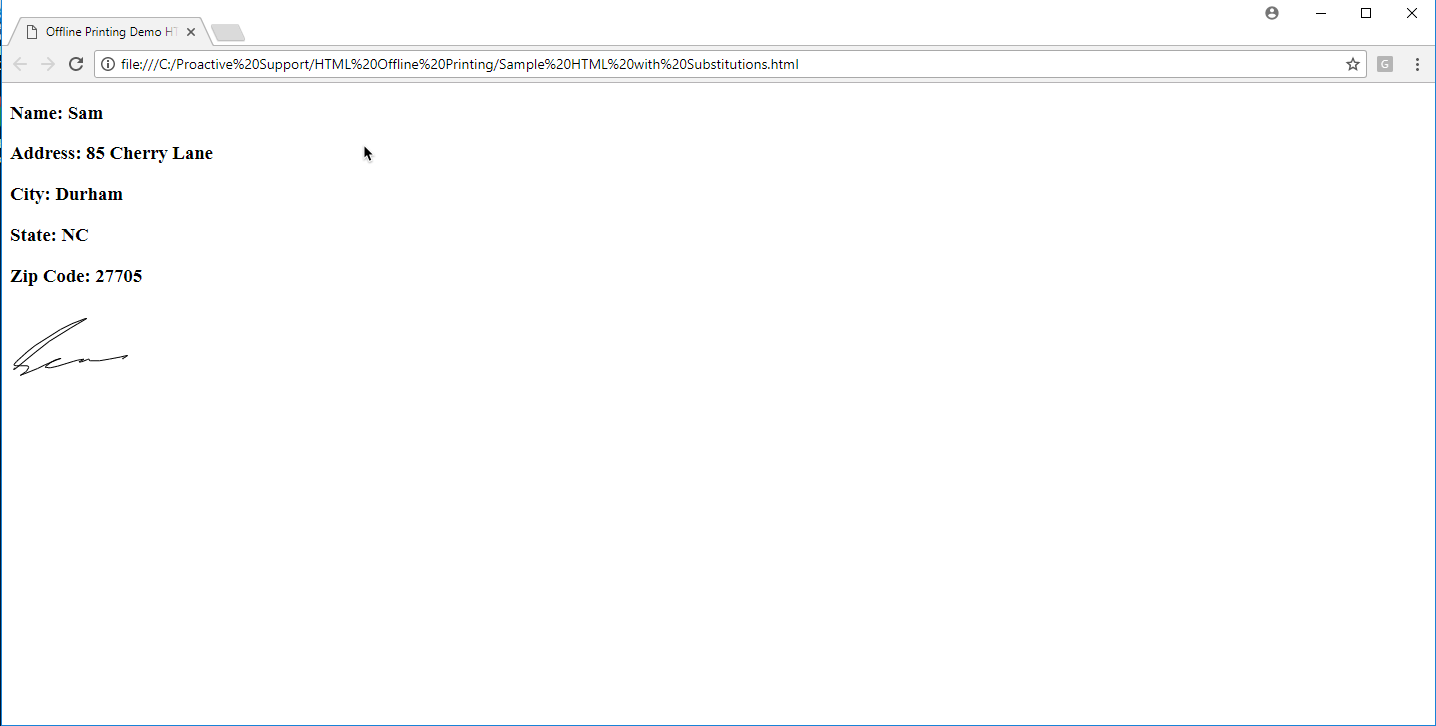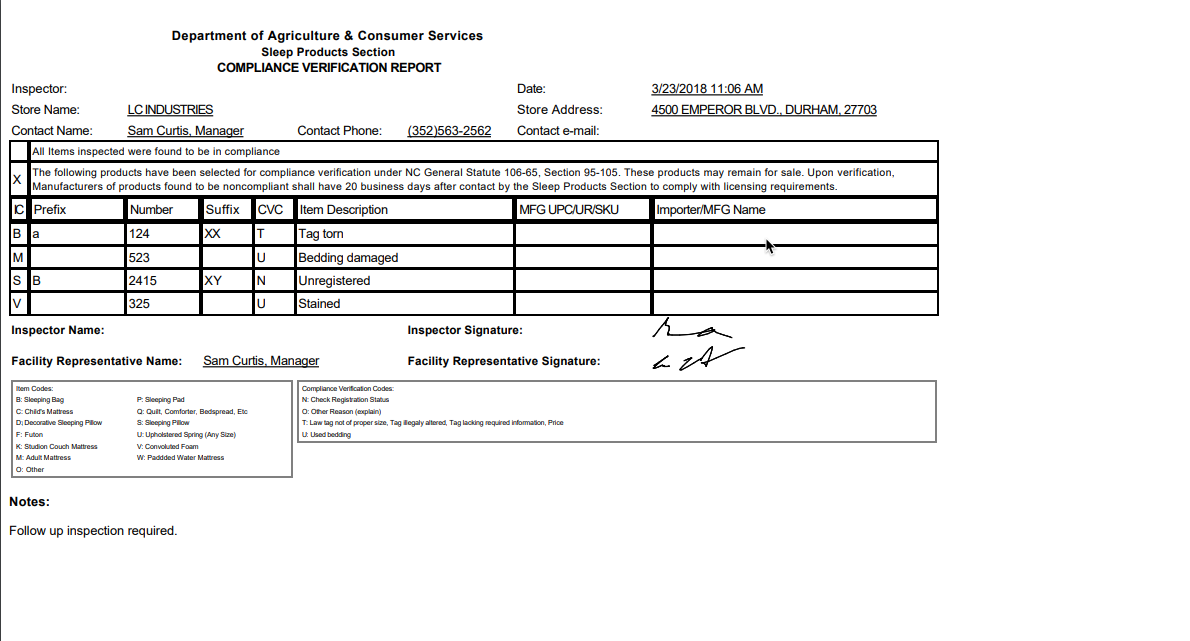In this example, we have a demo form that allows a user to enter their address data and then sign it to verify that it is correct.If we want to provide a printout of this information, even when the user is offline, we can do this using HTML and form script. Broadly, we place raw HTML into our form script as a string, and the use string substitution to place the field values from the form into the HTML, and then we can print out that HTML page.

In this example, let's assume we want our printed page to look like the photo below.The first thing we want to do is start with the raw HTML that creates a page formatted in the desired way. In this case, we are just using some bare-bones HTML with header fields to display each part of the information. But, this same approach will work for even highly complex HTML pages. Next, wherever in that HTML you want the values of your fields to show up, place some placeholder text that is easily identifiable. In this case, I have used the relevant field name surrounded by brackets. For example, I want whatever name the user enters to show up next to the text “Name:”, so I have placed  "name" in brackets in the appropriate spot. What this allows us to do is to use string substitution to substitute the values of the relevant fields for this placeholder text, resulting in our information being displayed correctly. Below is the HTML used for this example.

```<!DOCTYPE html>
<html>
<meta charset="UTF-8">
<title>Offline Printing Demo HTML</title>
<body>

<h3>Name: [Name]</h3>

<h3>City: [City]</h3>

<h3>State: [State]</h3>

<h3>Zip Code: [Zip]</h3>

<h3>[Signature]</h3>

</body>
</html>```

Then, create a string variable in form script with a value equal to the HTML you created. Be sure to properly handle quotation marks. If you use a single quote to enclose the string, make sure all quotation marks inside the string are double quotes, or vice versa. You can also escape quotation marks by converting " to \". Also, it is helpful to use an HTML minifier in order to condense the HTML into an easier string format.

`var rawHTML = '<!DOCTYPE html><html> <head> <meta charset=\"UTF-8\"> <title>Offline Printing Demo HTML</title> </head> <body> <h3>Name: [Name]</h3> <h3>Address: [Address]</h3> <h3>City: [City]</h3> <h3>State: [State]</h3> <h3>Zip Code: [Zip]</h3> <h3>[Signature]</h3> </body></html>';`

An action button will trigger the printing of our HTML. In this case, the field name of the print button is "PrintHTML", and I use the HotspotPressed event handler to trigger the call to my printHTML method.

```// Triggered when a hotspot is pressed
function EH_HotspotPressed(fieldName) {
if(fieldName == "PrintHTML"){
var htmlToPrint = getFormHTML();
}
}```

The printHTML function takes two arguments, the first is the HTML that is going to be printed, and the second is a title for the browser window that will open to print the HTML if the user is using Mi-Apps from a web browser. In order to get the field values to appear correctly in our HTML, we must substitute the values of the relevant fields into our HTML string variable. In this example, this is done using the getFormHTML method (see below). This method first uses the inkToSVG function to translate the signature field into an HTML svg element. The inkToSVG function takes two arguments, the first is the name of the relevant signature field, and the second is a scale factor. 40 is a good default value, but making that number smaller will shrink the size of the signature on the printout and making it bigger will increase the size of the signature on the printout. After handling the signature field, the getFormHTML method iterates through all the fields, and substitutes the fields values into the HTML string in the appropriate place, as identified by the placeholder tags we placed in the original HTML. Once the HTML contains the correct values, we can pass that HTML to the printHTML function, and the page will be printed.

```function getFormHTML(){
var fieldList = _form.getFieldList();
var formHTML = rawHTML;
var svg = inkToSVG("Signature", 40.0);
formHTML = formHTML.replace("[Signature]",svg);
for(var i=0; i<fieldList.length; i++){
var field = fieldList[i];
var fieldValue = _form.getValue(field);
formHTML = formHTML.replace("[" + field + "]", fieldValue);
}
return formHTML;
}

//utility function that prints the HTML contained in the html parameter
//if printing occurs in the browser, the title of the opened browser window will be specified by the title parameter
function printHTML(html, title){
if (!_form.platform.isWeb())
{
_form.printHTML(html);
}
else {
var myWindow=window.open('',title,'');
myWindow.document.write(html);
myWindow.document.close();
setTimeout(function() {
myWindow.focus();
myWindow.print();
}, 1000);
}
}

function inkToSVG(fieldName, multiplier)
{
if (_form.getInk == undefined)
{
return "";
}
var ink = _form.getInk(fieldName);
if (ink == null || ink.length == 0)
{
return "";
}
var strokes = ink.Strokes;
//var svg = '<svg>';
var minX = 100000000.0;
var maxX = 0;
var minY = 100000000.0;
var maxY = 0;
for (var i=0; i<strokes.length; i++)
{
var points = strokes[i].Points;
for (var j=0; j<points.length; j++)
{
if (points[j].X < minX)
{
minX = points[j].X;
}
if (points[j].Y < minY)
{
minY = points[j].Y;
}
if (points[j].X > maxX)
{
maxX = points[j].X;
}
if (points[j].Y > maxY)
{
maxY = points[j].Y;
}
}
}
minX *= 0.9;
minY *= 0.9;
maxX *= 1.1;
maxY *= 1.1;
var svg = '<svg height=\"' + maxY*multiplier + 'px\" width=\"' + maxX*multiplier +'px\">';

for (var i=0; i<strokes.length; i++)
{
var points = strokes[i].Points;
for (var j=1; j<points.length; j++)
{
svg += '<line x1=\"' + (points[j-1].X-minX)*multiplier + '\" y1=\"' + (points[j-1].Y-minY)*multiplier + '\" x2=\"' + (points[j].X-minX)*multiplier + '\" y2=\"' + (points[j].Y-minY)*multiplier + '\" style=\"stroke:rgb(0,0,0);stroke-width:1\" />';
}
}
svg += "</svg>";
return svg;
}```

This is a simple form, but this approach is a powerful method for creating printed reports of forms even when the user is offline. For example, below is a more complicated print out that involves displaying a table.The HTML and Mi-Apps form used in this example are attached to this article for reference.